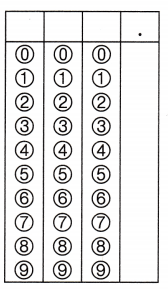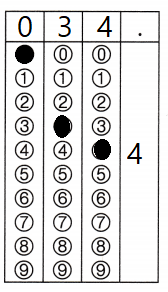Refer to our Texas Go Math Grade 3 Answer Key Pdf to score good marks in the exams. Test yourself by practicing the problems from Texas Go Math Grade 3 Module 5 Assessment Answer Key.

Vocabulary

Choose the best term from the box to complete the sentence.

Question 1.
Addition and subtraction are opposite operations, or ____. (p. 145)
Explanation:
Compatible numbers are pairs of numbers that are easy to add, subtract, multiply, or divide mentally.

Concepts and Skills

Use rounding or compatible numbers to estimate the difference. TEKS 3.4.B

Question 2.Question 4.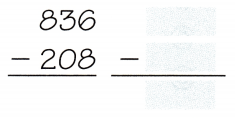Estimate. Find the difference. Then add to check. TEKS 3.4.A

Fill in the bubble for the correct answer choice.

Question 5.
Estimate: ____Explanation:
Estimate: 530 – 400 = 130
The difference is again added to verify

Go Math Module 5 Assessment Grade 3 Pdf Question 6.
Estimate: ____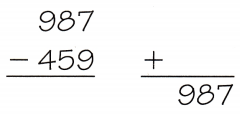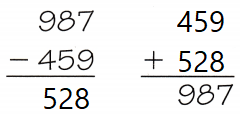Explanation:
Estimate: 1000 – 500 = 500
The difference is again added to verify

Question 7.
Estimate: ____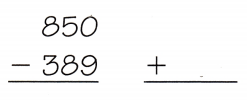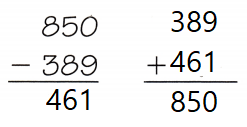Explanation:
Estimate: 800 – 300= 500
The difference is again added to verify

Estimate. Then find the difference. TEKS 3.4.A, 3.4.B

Question 8.
Estimate: ___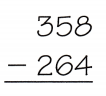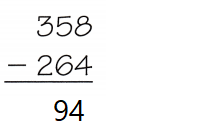Explanation:
Estimate: 350 – 250 = 100
The difference is 94

Question 9.
Estimate: ___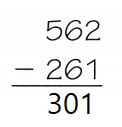Explanation:
Estimate: 500 – 200 = 300
The difference is 301

Estimate: ___Explanation:
Estimate: 800 – 500 = 300
The difference is 252

Question 10.
Estimate: ___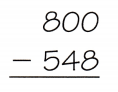Explanation:
Estimate: 800 – 500 = 300
The difference is 252

Question 11.
Estimate: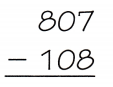Explanation:
Estimate: 800 – 100= 700
The difference is 699

Texas Test Prep

Fill in the bubble for the correct answer choice.

Question 12.
Jana has 147 rose bushes in her garden. She moves 119 of them to another garden. Which is the best estimate for the number of rose bushes she has left? TEKS 3.4.B
Explanation:
150 – 120 = 130
is the best estimate for the number of rose bushes she has left

Texas Go Math Grade 3 Answer Key Pdf Module 5 Question 13.
Lee is going to visit his grandmother. The car trip takes 56 minutes. If he stops for a snack after 17 minutes, how much time is left for the trip? TEKS 3.4.A, 3.4.B
(A) 40 minutes
(B) 30 minutes
(C) 10 minutes
(D) 20 minutes
Explanation:
60 – 20 = 40 minutes time is left for the trip

Question 14.
Angel’s school is having a used book sale. Their goal is to sell 600 books. They sold 240 books last week. This week they have sold 297 books. How many more books does the school need to sell to reach its goal? jy TEKS 3.4.A
(A) 537
(B) 163
(C) 63
(D) 297
Explanation:
240 + 297 = 537
600 – 537 = 63 more books does the school need to sell to reach its goal